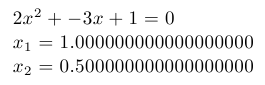Example for versions Web2c 2009

This example uses TeX fixed point arithmetic package fp and it’s macro for solving quadratic equations called `\FPqsolve`. Note that this macro can find only real roots of equation, and prints “FP error: Quadratic equation does not have a solution” if roots are complex (i.e., non-existent from its point of view).

``````\input fp.tex

\message{A = }
\message{B = }
\message{C = }

\FPqsolve{\xone}{\xtwo}{\number\a}{\number\b}{\number\c}

\$\a x^2+\b x+\c=0\$

\$x_1=\xone\$

\$x_2=\xtwo\$
\bye
``````Quadratic equation: document generated by TeX example program